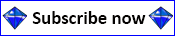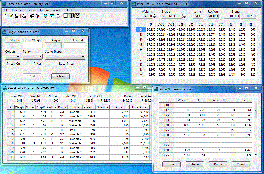## Sample Prices

The samples on this page cover the 3 month prices for 1.75ct - 1.99ct and 2.00ct - 2.24ct rough diamonds.

We currently publish four price lists for rough diamonds.

Our most commonly used list, it consists of 16 pages of prices covering weights from 0.10ct to 49.99ct for gem quality rough diamonds ie. colours D - M and purities IF - I3.

Rough diamonds falling into a specific weight range and shape are priced in a matrix of colour and purity (see sample page by clicking on the image.) Prices are displayed in tens of US Dollars per carat.

The evaluation procedure is as follows:

1. Weigh the rough diamond
2. Select the corresponding page based on the weight of the rough diamond (1)
3. Decide on the pricing section by determining the shape of the rough diamond
4. Decide on the colour and purity of the rough diamond
5. Look up the value in the pricing section (3) by finding where the colour row and purity column intersect
6. Multiply this value (5) by 10 to give you the US\$/ct price
7. Multiply the US\$/ct price (6) by the rough weight (1) to give you a "reasonable" price for the rough diamond

Generally, each stone is evaluated separately but if you have several stones with the same characteristics in the same weight range, you can evaluate them as a group.

A more detailed list of instructions with examples is available here.This price list tends to be used to give a rough idea of the value of a large parcel of rough diamonds where it is not desirable or necessary to evaluate each stone individually.

Basically, certain colours and purities have been grouped together and the simple arithmetic average of the individual classifications has been calculated. For example, prices for colours D and E having purities IF, VVS1 and VVS2 have been added together and divided by 6 to get to the value displayed in the list. However, inaccuracies occur when the number of poorer quality stones in a grouping aren't matched by the number of better stones.

The evaluation procedure is as follows:

1. Sort the rough diamonds into the different weight categories
2. Sort each weight category (1) into the shape, colour and purity groups
3. Select the corresponding page for the selected weight category (1)
4. Look up the value corresponding to the shape, colour and purity (2) group
5. Multiply this value (4) by 10 to give you the US\$/ct price
6. Multiply the US\$/ct price (5) by the total rough weight of the group to give you a rough price for the rough diamondsThis price list gives a better idea of the value of a large parcel of rough diamonds than the Average Guideline Price List because it tries to approximate the distribution of colours and purities in a run-of-mine production.

Colours and purities have been grouped together in such a way that twice as many stones are assumed to be in the purity to the right of a given stone, and twice as many stones are assumed to be in the colour below a given stone. For example, prices for colours D and E having purities IF, VVS1 and VVS2 have been added together as (1 x D-IF) + (2 x D-VVS1) + (2 x E-IF) + (4 x D-VVS2) + (4 x E-VVS1) + (8 x E-VVS2) and divided by 21 to get to the value displayed in the list. This should provide a more accurate valuation than the Average Guideline Price List above.

The evaluation procedure is as follows:

1. Sort the rough diamonds into the different weight categories
2. Sort each weight category (1) into the shape, colour and purity groups
3. Select the corresponding page for the selected weight category (1)
4. Look up the value corresponding to the shape, colour and purity (2) group
5. Multiply this value (4) by 10 to give you the US\$/ct price
6. Multiply the US\$/ct price (5) by the total rough weight of the group to give you a rough price for the rough diamondsThis price list, developed in collaboration with Eddy Vleeschdrager (www.expert-diamond.com), provides prices for coloured rough diamonds ranging from 0.10ct to 49.99ct.

Because of the wide range of prices paid for coloured diamonds on the open market, we have provided a low and a high price for each stone category. Because colour is a very important characteristic, not too much emphasis is placed on purity. Therefore, purities are grouped into visually clean, slightly included and included 1, 2 and 3 categories.

The evaluation procedure is as follows:

1. Weigh the rough diamond
2. Select the corresponding pages (5) based on the weight of the rough diamond (1)
3. Decide on the pricing page (from the 5 pages in step 2) by determining the shape of the rough diamond
4. Decide on the colour and purity of the rough diamond
5. Look up the values (low and high) in the pricing section (3) by finding where the colour row and purity column intersect
6. Multiply these values (5) by 10 to give you the US\$/ct prices
7. Multiply the US\$/ct prices (6) by the rough weight (1) to give you a range of prices you can pay for the rough diamond

Because coloured diamonds are thinly traded, you should try to purchase at the lower end of the indicated price range to reduce your risk.Rough Diamond Parcel Calculator

For those who don't like doing things manually, we have a Rough Diamond Parcel Calculator software program that uses an electronic version of the Rough Diamond Guideline Price List to calculate the value of a parcel of rough diamonds; it therefore only works for gem-quality rough diamonds.

It is Windows-based, and works on both 32-bit and 64-bit versions of the operating system. A fully functional demonstration version is available for you to evaluate its functionality.

Usage is similar to any Windows-based program. You enter the characteristics of the rough diamond and the program displays the guideline price. You have the ability to override the suggested price as you see fit.

There is online help which has a number of suggestions as well as instructions on how to use the various parts of the program. Check out the demonstration version for details.